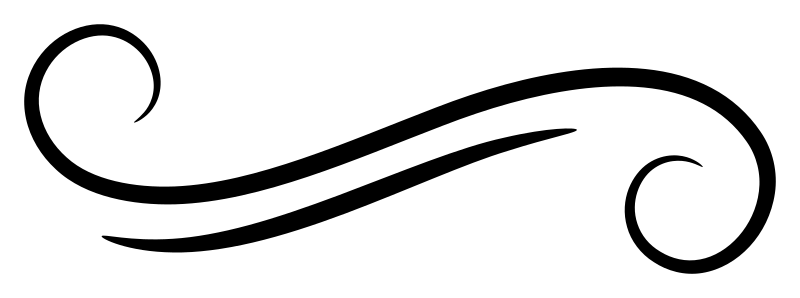# Featured functions

Featured function pages are quick overviews of interesting functions we just want to draw more attention to.

• (\$)
• `(\$) :: (a -> b) -> a -> b`
• intercalate
• `intercalate :: [a] -> [[a]] -> [a]`
• mapMaybe
• `mapMaybe :: (a -> Maybe b) -> [a] -> [b]`
• null
• `null :: Foldable t => t a -> Bool`
• Rounding
• `round :: (RealFrac a, Integral b) => a -> b`
• `truncate :: (RealFrac a, Integral b) => a -> b`
• `ceiling :: (RealFrac a, Integral b) => a -> b`
• `floor :: (RealFrac a, Integral b) => a -> b`
• singleton
• `singleton :: a -> [a]`
• toIntegralSized
• `toIntegralSized :: (Integral a, Integral b, Bits a, Bits b) => a -> Maybe b`Join Type Classes for courses and projects to get you started and make you an expert in FP with Haskell.